# Trade Math for the HVACR Service Engineer

Throughout your career as an HVACR technician, you will find yourself relying on specific equations to interpret readings taken with your test instruments. Some equations apply particularly to hydronic systems, others to air distribution, others to electrical troubleshooting, and so on. Listed below are some equations commonly used in the HVACR trade:

• Sensible heat equation: Btuh (sensible) = 1.08 x cfm x ΔT
• Latent heat equation: Btuh (latent) = 0.68 x cfm x Δgrains
• Total heat equation: Btuh (total) = 4.5 x cfm x Δenthalpy
• Converting watts to Btuh: Btuh = W x 3.413
• Ohm’s Law: E = I x R
• Power equation: P = E x I
• Resistors in series: Rtotal = R1 + R2 + R3 + …Rn
•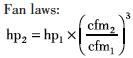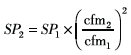•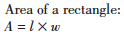•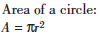•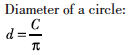•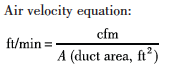You should be familiar with most of the symbols and abbreviations used in these equations. Some of the more common ones include:

• A = area (in2, ft2, m2, cm2)
• C = circumference (in, ft, m, cm)
• C = capacitance (μF)
• cfm = cubic feet per minute (volume)
• d = diameter (in, ft, m, cm)
• E = voltage (volts)
• ft/min = feet per minute (velocity)
• hp = horsepower
• I = current (amperes)
• l = length
• P = power
• R = resistance (ohms)
• SP = static pressure (in. w.g.)
• T = temperature (°F, °C)
• W = watts
• w = width
• D = delta or difference
• p = pi (3.14)

There are many more equations used to evaluate the operating performance of HVACR systems than those listed above. Mastering the equations shown here, however, will give you a good foundation.

Excerpted from Preparing for the NATE Exam: Core Essentials, published by RSES.

For more essential study materials visit the online store at the Refrigeration Service Engineers Society.

Scroll to Top